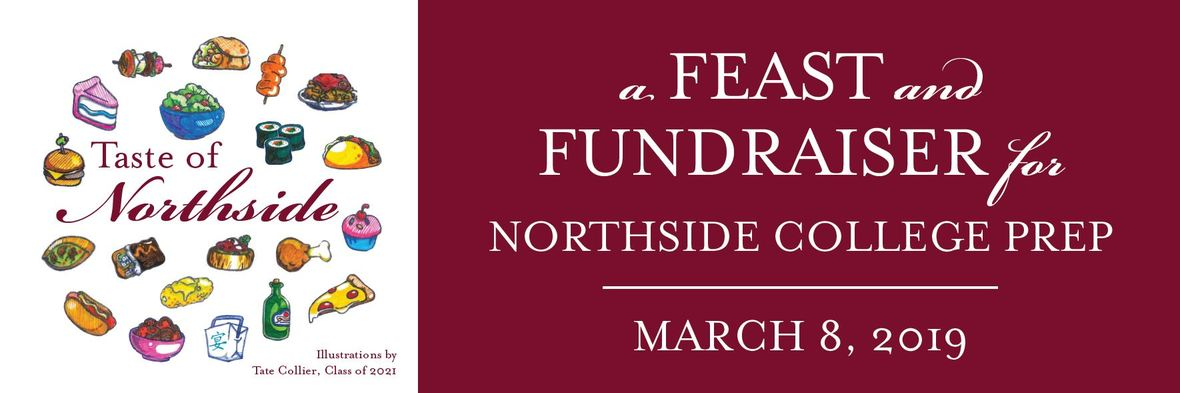# Don't Sit on the Fence!

\$95 early bird online ticket sales close at 11:59 pm TODAY!

 table.module-4{width:41.13%;padding:0}table div table+table+table+table+table div table{width:41.13%;float:none;margin-left:auto;margin-right:auto;padding:0}table div table+table+table+table+table div table a{border:0 none;text-decoration:none}table div table+table+table+table+table div table img{width:100%!important;border:0 none;text-decoration:none}table div table+table+table+table+table div table td{width:100%;padding:0}/* styles */

# Taste of Northside FAQ's

## Why attend?

Join fellow Northside parents, alumni, and faculty for a fun, casual evening in one of the city’s historic venues close to school. Your ticket includes a dinner buffet with an open bar, and live music. Attendance at the Taste also gives you exclusive access to bid on the live auction items.

 /* styles */ The Spring Benefit is an opportunity for everyone to help ensure that Northside has the critical resources it needs to remain one of the nation's leading high schools. Donate an item, volunteer, bid on an item, or attend the Taste. We can't do it without your participation!

CPS budget challenges make your financial support crucial. Purchasing tickets at the Sponsorship level means more funds go directly to sustaining the quality of education for our children.

## Will there be food?

Not only will there be food, but there will be food from some of Chicagoland's best restaurants! We have a delicious, complete dinner buffet menu planned, with tastes from these restaurants:## Will there be drinks?

Yes! Your ticket includes an open bar, featuring beer from Lagunitas and Revolution Brewing, wines from Vin Chicago, and a specialty cocktail with Wheatley Vodka.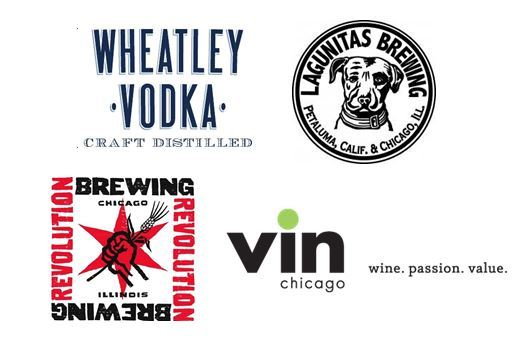## Do I have to dress up?

No! This is a casual, relaxed evening about supporting Northside, not about fancy clothes. What is the dress code? Anything goes!

## Is there entertainment at the Taste of Northside?

Absolutely! Guests who purchase tickets at a Sponsorship level will begin their evening with the sweet sounds of music by members of Northside's very own student chorus.

As the Taste begins, all guests will enjoy music led by NCP dad Matt Farmer, with Steve Doyle and Lisa DeRosia.

Finally, we'll move into the live auction with our very popular, returning emcee, Mr. William Nifong!

## Tell me about the auction.

The Silent Auction will go live, online, on March 1. Bid for the opportunity to win sports tickets, restaurant gift certificates, unique experiences, and more!

At the Taste, you'll be able to make contributions to the fund-a-need, purchase raffle tickets, bid on silent and live auction items, and raise your paddle to support the Better Library, Brighter Future campaign to raise money to refurbish the NCP Library.

## What about sweets? We need dessert!

There will be a dessert buffet featuring Lucila's Alfajores and Eli's Cheesecake!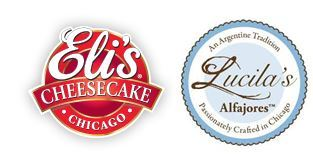## How do we get there?

The DANK Haus is conveniently located in Lincoln Square, half block north of the Western Brown Line station. Guests are welcome to park in the MB Financial parking lot at Western & Gunnison Avenue. Paid street parking is available along Western Avenue with two parking lots located just north of the Western Brown Line Stop on the east side of Western Avenue (via Leland Avenue). The location is also easily accessible by public transit.

CTA: Brown Line Western Stop, Bus #49 Western, Bus #X49 Western Express, Bus #81 Lawrence
Metra: Union Pacific North Line - Ravenswood

 table div table+table+table+table+table+table+table+table+table+table+table+table+table+table+table+table+table+table+table+table div table{width:100%;padding:0}table div table+table+table+table+table+table+table+table+table+table+table+table+table+table+table+table+table+table+table+table div table img{width:96.23%;padding:0;float:none}table div table+table+table+table+table+table+table+table+table+table+table+table+table+table+table+table+table+table+table+table div table td{width:100%;padding:0 1.88% 18px}/* styles */Please volunteer for a shift in support of our annual benefit. View and sign up for open positions HERE.

 table div table+table+table+table+table+table+table+table+table+table+table+table+table+table+table+table+table+table+table+table+table+table div table{width:100%;padding:0}table div table+table+table+table+table+table+table+table+table+table+table+table+table+table+table+table+table+table+table+table+table+table div table img{width:96.23%;padding:0;float:none}table div table+table+table+table+table+table+table+table+table+table+table+table+table+table+table+table+table+table+table+table+table+table div table td{width:100%;padding:0 1.88% 18px}/* styles */# Auction Donations Still Needed!

It’s not too late to donate items to our silent auction. This major fundraiser raises critical funds to support Northside. Please help us gather items to include in our online silent auction.

Important Auction Dates:
Online Auction: March 1 - 10
Live Auction at the Party: March 8

Kristen Werries Collier, kristenwerriescollier@gmail.com.

You can drop off auction items at school or email the committee HERE. Deadline for submissions is February 26.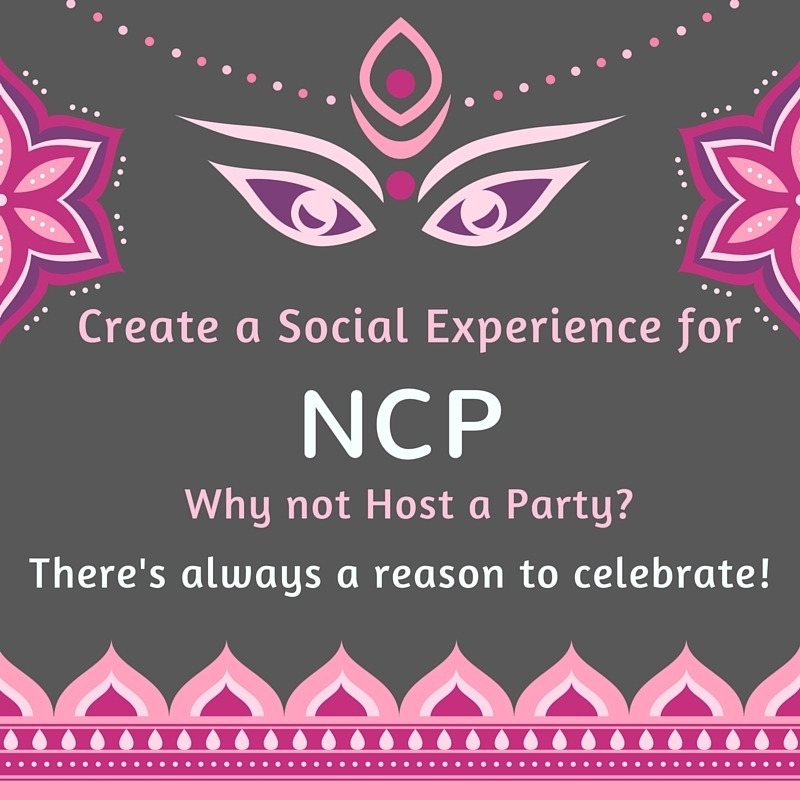## Build a Social Experience for NCP

A fun aspect of our annual Benefit fundraising effort each year is the Parent Parties! Northside families offer to host a party for other Northside parents and families. The host pays for the party, guests pay to attend, and the revenue generated from tickets is all donated to Northside. These parties raise \$500-\$2500 each and are a great opportunity to meet and mingle with other Northside parents. A few ideas are Moms & Margaritas, Poker Night, Wine Tasting, Backyard BBQ, Theme Dinner Party, and more.

Please consider hosting a party like one of those suggested, come up with your own idea, or we can help you put something together. Reach out to Nancy Liljedahl at n.liljedahl@comcast.net if you are interested in hosting or want to brainstorm. Thanks for your support!## The NCP ONLINE AUCTION Runs March 1 – 10

This is one of our most important fundraisers and lets you nab fabulous items donated by amazing businesses in the community. Your bids directly benefit our Northside students.

Here are just a few of this year’s auction items:

▪ Four 100-level Chicago Bulls tickets
▪ Front row seats to see Jeff Tweedy at the Vic
▪ Dinner for two with the Hungry Hound, Steve Dolinsky of ABC News
▪ Dremel 3D Printer
▪ Tooth whitening package with Dr. Baukol DDS
▪ Dinner for two at Frontera Grill, including cookbooks signed by chef Rick Bayless
▪ Three-night Tucson Getaway
 ▪ Four 100-level Chicago Bulls tickets
 ▪ Front row seats to see Jeff Tweedy at the Vic
 ▪ Dinner for two with the Hungry Hound, Steve Dolinsky of ABC News
 ▪ Dremel 3D Printer
 ▪ Tooth whitening package with Dr. Baukol DDS
 ▪ Dinner for two at Frontera Grill, including cookbooks signed by chef Rick Bayless
 ▪ Three-night Tucson Getaway

Set your calendar to start bidding on March 1. We’re still taking donations for the auction too!We are putting together a slideshow of our students participating in the many activities that make Northside special. If you have pictures you'd like to be considered for inclusion in the presentation, please forward them to Debra Swan at debra@theswans.net.

# Thank You to our Corporate Sponsors!

Corporate Sponsorship contributions help underwrite the cost of the 2019 Spring Benefit and direct funds raised at the Taste directly to programs and services for the school not covered by the CPS budget. It's not too late to be a corporate sponsor. Please contact Matt Schechter, at matthew.d.schechter@gmail.com. Click HERE for the sponsorship form.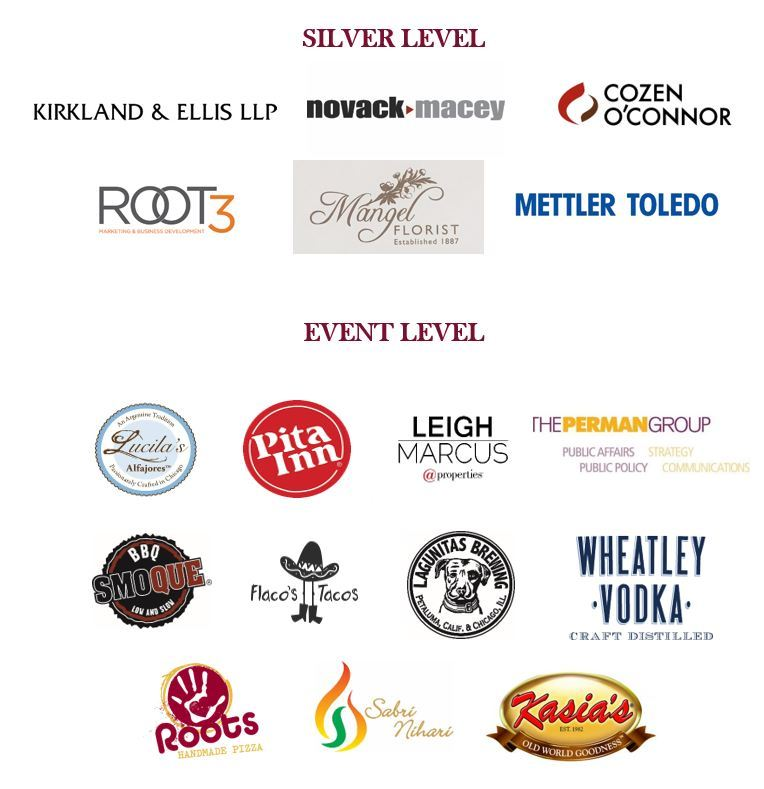table div table+table+table+table+table+table+table+table+table+table+table+table+table+table+table+table+table+table+table+table+table+table+table+table+table+table+table+table+table div table{width:100%;padding:0}table div table+table+table+table+table+table+table+table+table+table+table+table+table+table+table+table+table+table+table+table+table+table+table+table+table+table+table+table+table div table img{width:96.23%;padding:0;float:none}table div table+table+table+table+table+table+table+table+table+table+table+table+table+table+table+table+table+table+table+table+table+table+table+table+table+table+table+table+table div table td{width:100%;padding:0 1.88% 18px}/* styles */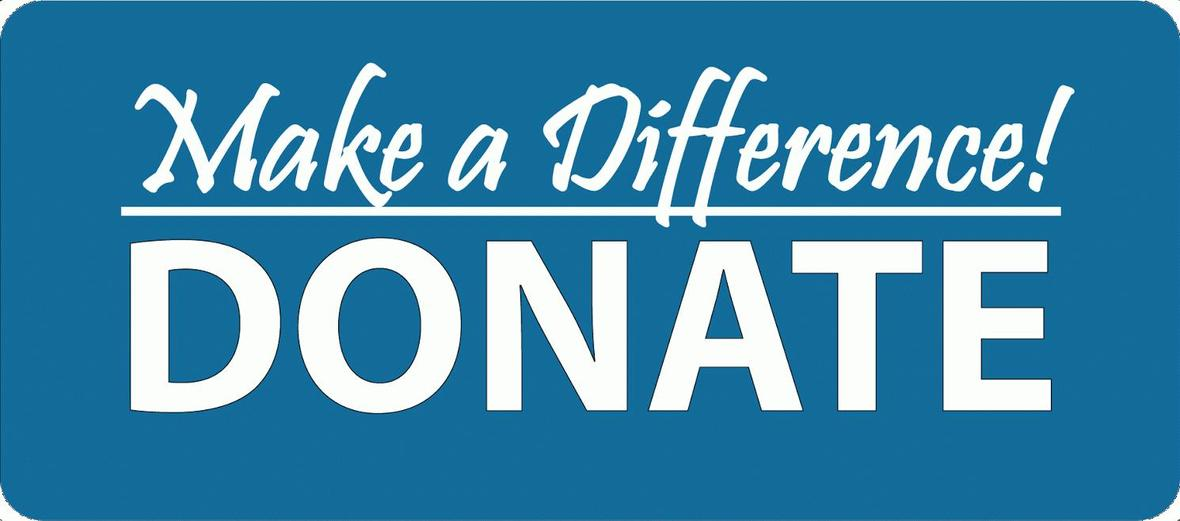# Student Resource Closet

## Donations Needed

Friends of Northside has a resource closet for our NCP students. We would like to fill it with school supplies, non-perishable food items, hygiene products, and clothing necessities.
All items can be brought into the counseling office and placed in the large bin marked “Student Supply Box.”

Please make sure all of the items are new and unopened. No used clothing please.

Thanks for your help and support!

Supply List:

▪ “New” winter gloves for men and women
▪ Snacks like granola bars, nuts, and dried fruit
 ▪ “New” winter gloves for men and women
 ▪ Snacks like granola bars, nuts, and dried fruit

Please bring your items into the Counseling Office and put into the gray bin.

 table div table+table+table+table+table+table+table+table+table+table+table+table+table+table+table+table+table+table+table+table+table+table+table+table+table+table+table+table+table+table+table div table{width:100%;padding:0}table div table+table+table+table+table+table+table+table+table+table+table+table+table+table+table+table+table+table+table+table+table+table+table+table+table+table+table+table+table+table+table div table img{width:96.23%;padding:0;float:none}table div table+table+table+table+table+table+table+table+table+table+table+table+table+table+table+table+table+table+table+table+table+table+table+table+table+table+table+table+table+table+table div table td{width:100%;padding:0 1.88% 18px}/* styles */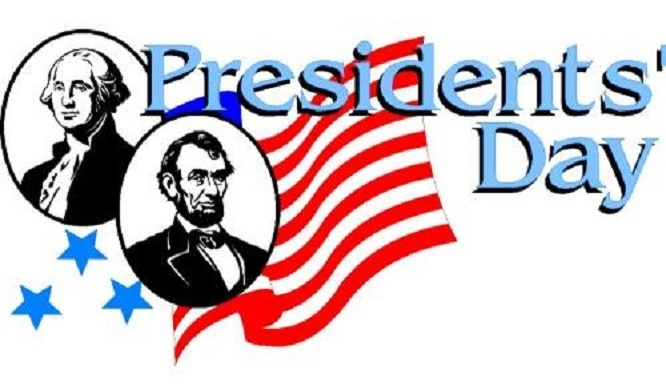# No School

Monday, February 18
Presidents' Day

 table div table+table+table+table+table+table+table+table+table+table+table+table+table+table+table+table+table+table+table+table+table+table+table+table+table+table+table+table+table+table+table+table+table div table{width:100%;padding:0}table div table+table+table+table+table+table+table+table+table+table+table+table+table+table+table+table+table+table+table+table+table+table+table+table+table+table+table+table+table+table+table+table+table div table img{width:96.23%;padding:0;float:none}table div table+table+table+table+table+table+table+table+table+table+table+table+table+table+table+table+table+table+table+table+table+table+table+table+table+table+table+table+table+table+table+table+table div table td{width:100%;padding:0 1.88% 18px}/* styles */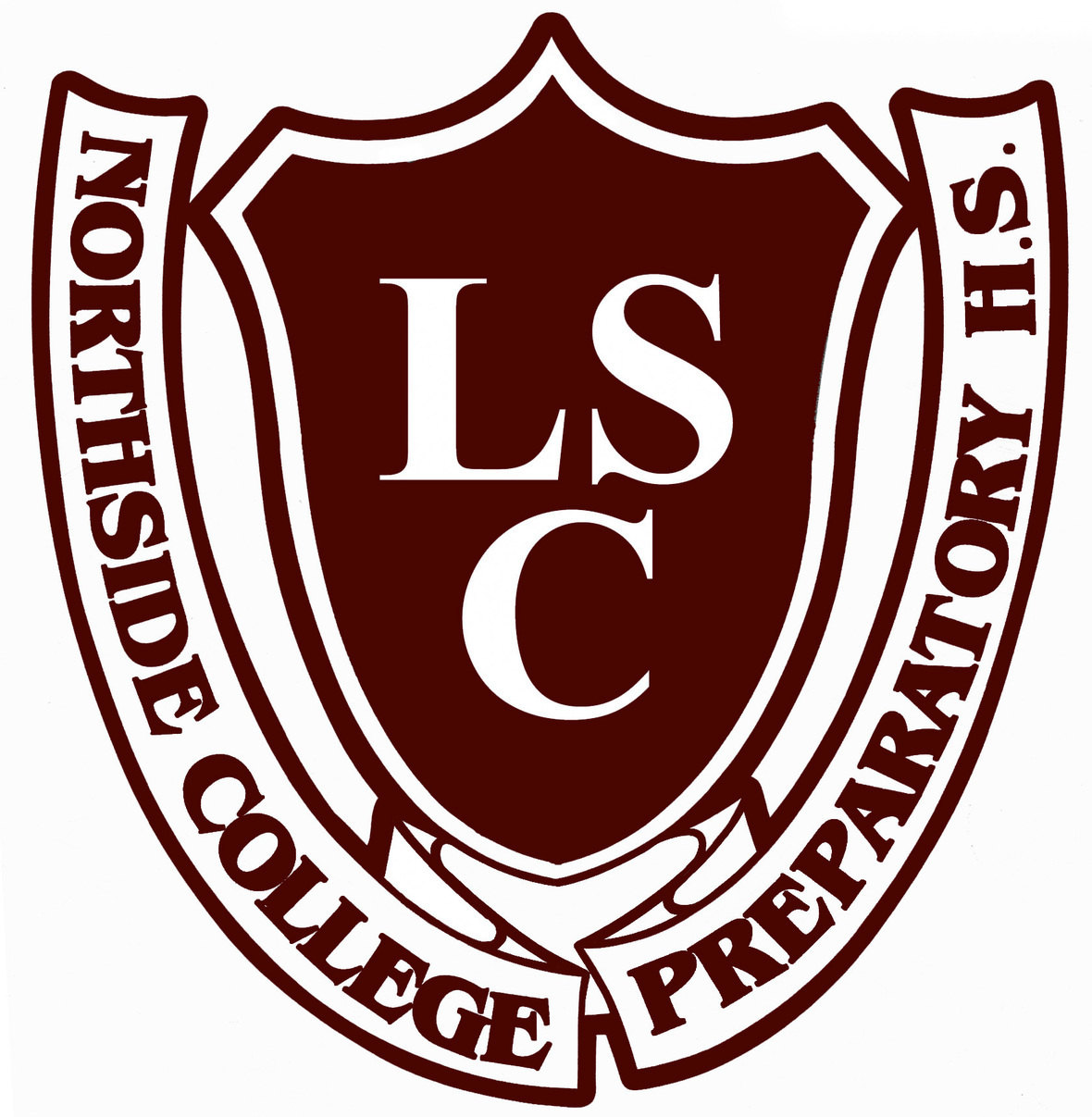# LSC Meeting

Tuesday, February 19
6:30 pm
NCP Faculty Lounge

 table div table+table+table+table+table+table+table+table+table+table+table+table+table+table+table+table+table+table+table+table+table+table+table+table+table+table+table+table+table+table+table+table+table+table+table div table{width:100%;padding:0}table div table+table+table+table+table+table+table+table+table+table+table+table+table+table+table+table+table+table+table+table+table+table+table+table+table+table+table+table+table+table+table+table+table+table+table div table img{width:96.23%;padding:0;float:none}table div table+table+table+table+table+table+table+table+table+table+table+table+table+table+table+table+table+table+table+table+table+table+table+table+table+table+table+table+table+table+table+table+table+table+table div table td{width:100%;padding:0 1.88% 18px}/* styles */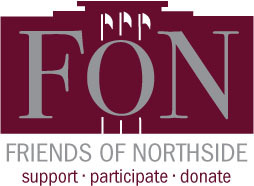## Become a FON Board Member

Friends of Northside (FON) is a parent organization that works to support the Northside community through fundraising and volunteerism. All parents and guardians are automatically members of FON. Board positions are available for those who would like to take a more active role.

FON's fundraising seeks to raise money with the goals of replacing lost funding from CPS, supporting the school’s mission, and maintaining and enhancing the learning experience for all NCP students. FON efforts include the Annual Appeal, Spring Benefit, and Cubby Walk Walkathon, among others.

FON also provides volunteers and hospitality to the NCP community. It plans social/educational programs, recruits volunteers for various NCP events such as Open House, distributes this weekly email communication, and maintains the student directory.

## Come see what we're all about!

Tuesday, February 26, 7:00 pm
Faculty Lounge

 table div table+table+table+table+table+table+table+table+table+table+table+table+table+table+table+table+table+table+table+table+table+table+table+table+table+table+table+table+table+table+table+table+table+table+table+table+table div table{width:100%;padding:0}table div table+table+table+table+table+table+table+table+table+table+table+table+table+table+table+table+table+table+table+table+table+table+table+table+table+table+table+table+table+table+table+table+table+table+table+table+table div table img{width:96.23%;padding:0;float:none}table div table+table+table+table+table+table+table+table+table+table+table+table+table+table+table+table+table+table+table+table+table+table+table+table+table+table+table+table+table+table+table+table+table+table+table+table+table div table td{width:100%;padding:0 1.88% 18px}/* styles */# College Planning Night for Juniors

Thursday, February 28
6:00 - 7:30 pm
Auditorium

Tambien tendremos una sección en Español.
Rowniez mamy sesje w jezyku Polskim.

Northside Prep Counseling Department is looking forward to seeing all junior students and their parents/guardians on Thursday, February 28, for the annual College Planning Night. The counseling team will share an overview of the planning steps for the college admission process, NCP application process, post-secondary resources, and information to assist your family with scheduling a college conference. Also, students will receive access to the College Planning Handbook and parents will receive their registration code for Naviance, our college application management program.

Both students and parents are highly encouraged to attend because this is a milestone that will assist your family with successfully navigating the college application process! Our goal is 100% attendance by juniors and their parents/guardians. This is an exciting time in your life and the NCP counselors look forward to helping you with your personal journey.

If you have any questions or concerns, contact your respective counselor.

 table div table+table+table+table+table+table+table+table+table+table+table+table+table+table+table+table+table+table+table+table+table+table+table+table+table+table+table+table+table+table+table+table+table+table+table+table+table+table+table div table{width:100%;padding:0}table div table+table+table+table+table+table+table+table+table+table+table+table+table+table+table+table+table+table+table+table+table+table+table+table+table+table+table+table+table+table+table+table+table+table+table+table+table+table+table div table img{width:96.23%;padding:0;float:none}table div table+table+table+table+table+table+table+table+table+table+table+table+table+table+table+table+table+table+table+table+table+table+table+table+table+table+table+table+table+table+table+table+table+table+table+table+table+table+table div table td{width:100%;padding:0 1.88% 18px}/* styles */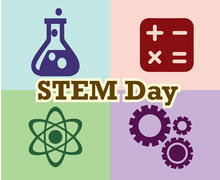# STEM Day 2019 - Parent Volunteers Needed!

Saturday, March 16

The event, spearheaded by students, is geared towards elementary-age children. Northside students will have interactive presentations on STEM-related topics for children to partake in.

Adult volunteers are needed for two areas:
1) Mentor Northside students with presentations.
2) Provide logistical support to the Planning Committee (e.g. securing food and other materials) and acting as ushers and guides on the actual day.

All volunteers must be Level II CPS Volunteers.

 table div table+table+table+table+table+table+table+table+table+table+table+table+table+table+table+table+table+table+table+table+table+table+table+table+table+table+table+table+table+table+table+table+table+table+table+table+table+table+table+table+table div table{width:100%;padding:0}table div table+table+table+table+table+table+table+table+table+table+table+table+table+table+table+table+table+table+table+table+table+table+table+table+table+table+table+table+table+table+table+table+table+table+table+table+table+table+table+table+table div table img{width:96.23%;padding:0;float:none}table div table+table+table+table+table+table+table+table+table+table+table+table+table+table+table+table+table+table+table+table+table+table+table+table+table+table+table+table+table+table+table+table+table+table+table+table+table+table+table+table+table div table td{width:100%;padding:0 1.88% 18px}/* styles */## Purchase Yearbook Personal Ads for Seniors

All materials are due by Friday, March 17.

Commemorate your student's accomplishments or declare your best wishes with a personal ad in the upcoming Stampede. The prices are:

▪ Full Page \$150
▪ Half Page \$80
▪ Quarter Page \$50

Members of the Stampede can create the ad for you, or you can submit a pre-made ad yourself (photo resolution no less than 600 dpi, please). If you choose to submit a pre-made ad, please also email the digital photos as separate attachments.

Questions? Contact Marion McCreedy, Stampede adviser, at mkmccreedy@cps.edu.## And While We're Talking About Yearbooks...

Order yours now to avoid the March 1 price increase!

Yearbooks were not included as an item on the school fee schedule this year.

2) Enter code 4187219

 table div table+table+table+table+table+table+table+table+table+table+table+table+table+table+table+table+table+table+table+table+table+table+table+table+table+table+table+table+table+table+table+table+table+table+table+table+table+table+table+table+table+table+table+table+table div table{width:100%;padding:0}table div table+table+table+table+table+table+table+table+table+table+table+table+table+table+table+table+table+table+table+table+table+table+table+table+table+table+table+table+table+table+table+table+table+table+table+table+table+table+table+table+table+table+table+table+table div table img{width:96.23%;padding:0;float:none}table div table+table+table+table+table+table+table+table+table+table+table+table+table+table+table+table+table+table+table+table+table+table+table+table+table+table+table+table+table+table+table+table+table+table+table+table+table+table+table+table+table+table+table+table+table div table td{width:100%;padding:0 1.88% 18px}/* styles */# Juniors - Class of 2020! Save the Dates!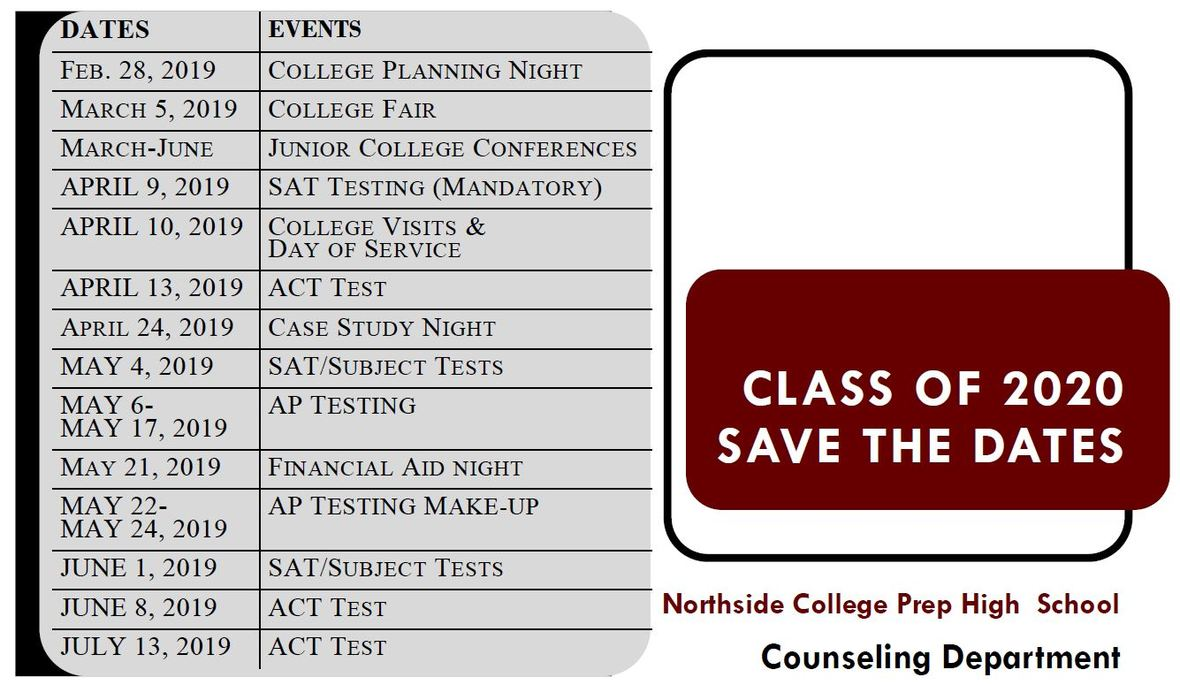table div table+table+table+table+table+table+table+table+table+table+table+table+table+table+table+table+table+table+table+table+table+table+table+table+table+table+table+table+table+table+table+table+table+table+table+table+table+table+table+table+table+table+table+table+table+table+table+table div table{width:100%;padding:0}table div table+table+table+table+table+table+table+table+table+table+table+table+table+table+table+table+table+table+table+table+table+table+table+table+table+table+table+table+table+table+table+table+table+table+table+table+table+table+table+table+table+table+table+table+table+table+table+table div table img{width:96.23%;padding:0;float:none}table div table+table+table+table+table+table+table+table+table+table+table+table+table+table+table+table+table+table+table+table+table+table+table+table+table+table+table+table+table+table+table+table+table+table+table+table+table+table+table+table+table+table+table+table+table+table+table+table div table td{width:100%;padding:0 1.88% 18px}/* styles */Ms. Woodruff is hoping to run a sewing colloquium this spring but needs sewing machines. If you have a sewing machine you can donate, please contact Ms. Woodruff at jwoodruff@cps.edu.

Thank you to everyone who has donated a sewing machine. And thank you to SewVacDirect who donated 2 brand-new machines!

 table div table+table+table+table+table+table+table+table+table+table+table+table+table+table+table+table+table+table+table+table+table+table+table+table+table+table+table+table+table+table+table+table+table+table+table+table+table+table+table+table+table+table+table+table+table+table+table+table+table+table div table{width:100%;padding:0}table div table+table+table+table+table+table+table+table+table+table+table+table+table+table+table+table+table+table+table+table+table+table+table+table+table+table+table+table+table+table+table+table+table+table+table+table+table+table+table+table+table+table+table+table+table+table+table+table+table+table div table img{width:96.23%;padding:0;float:none}table div table+table+table+table+table+table+table+table+table+table+table+table+table+table+table+table+table+table+table+table+table+table+table+table+table+table+table+table+table+table+table+table+table+table+table+table+table+table+table+table+table+table+table+table+table+table+table+table+table+table div table td{width:100%;padding:0 1.88% 18px}/* styles */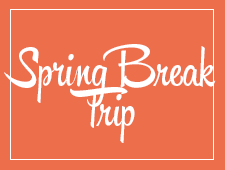# Travel this Spring with Northside

## Spring Break 2019 Trip to the Grand Canyon

Students will have a chance to connect with the majesty of our National Park system. We will spend 5 nights in the Grand Canyon camping, hiking, stargazing, and learning wilderness skills. Students will participate in a second-semester colloquium to prepare them for the trip. Trip sponsors and chaperones include Ms. Qazi, Ms. Schaldenbrand, and Mr. Barry Smith. Approximate cost: \$1,000 (includes flight, food, and lodging).

 table div table+table+table+table+table+table+table+table+table+table+table+table+table+table+table+table+table+table+table+table+table+table+table+table+table+table+table+table+table+table+table+table+table+table+table+table+table+table+table+table+table+table+table+table+table+table+table+table+table+table+table+table div table{width:100%;padding:0}table div table+table+table+table+table+table+table+table+table+table+table+table+table+table+table+table+table+table+table+table+table+table+table+table+table+table+table+table+table+table+table+table+table+table+table+table+table+table+table+table+table+table+table+table+table+table+table+table+table+table+table+table div table img{width:96.23%;padding:0;float:none}table div table+table+table+table+table+table+table+table+table+table+table+table+table+table+table+table+table+table+table+table+table+table+table+table+table+table+table+table+table+table+table+table+table+table+table+table+table+table+table+table+table+table+table+table+table+table+table+table+table+table+table+table div table td{width:100%;padding:0 1.88% 18px}/* styles */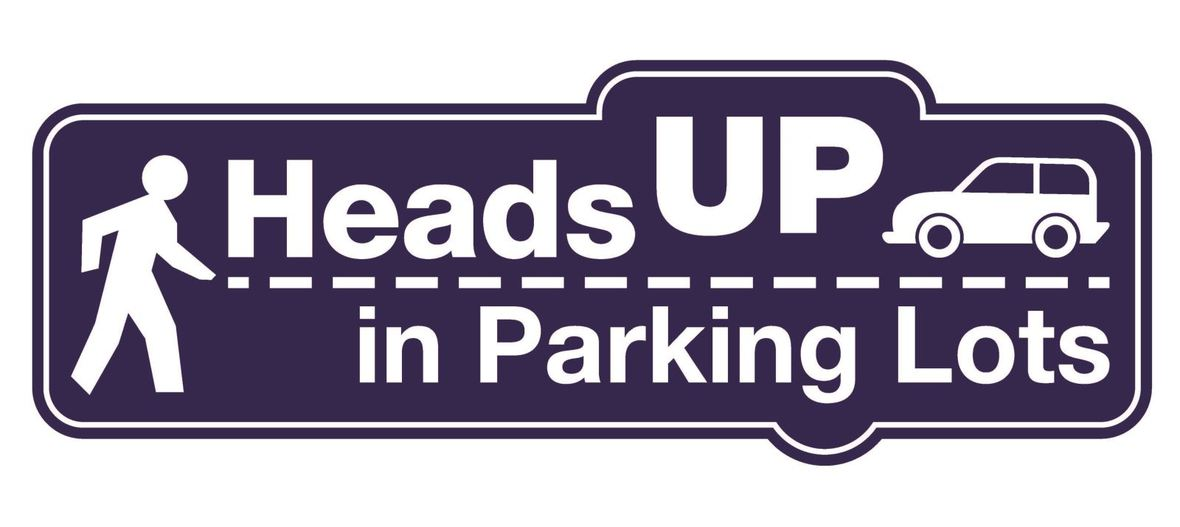# Parking Lot Safety and Procedures

Our NCP school parking lot is a busy place before and after school. Families dropping off and picking up students are asked to be mindful of both safety and courtesy. Please assist us by ensuring that traffic lanes stay clear during drop-off and pick-up times. Pulling over to the curb on the right as your student enters or exits your vehicle keeps students safe and traffic flow efficient.

Please remember that ALL VEHICLES MUST EXIT ONTO KEDZIE. You may enter the parking lot from Kedzie or Bryn Mawr, but regardless of where you enter, all cars must exit onto Kedzie. For the safety of our students and the rest of the Northside community, please do not exit onto Bryn Mawr.

 table div table+table+table+table+table+table+table+table+table+table+table+table+table+table+table+table+table+table+table+table+table+table+table+table+table+table+table+table+table+table+table+table+table+table+table+table+table+table+table+table+table+table+table+table+table+table+table+table+table+table+table+table+table+table+table div table{width:100%;padding:0}table div table+table+table+table+table+table+table+table+table+table+table+table+table+table+table+table+table+table+table+table+table+table+table+table+table+table+table+table+table+table+table+table+table+table+table+table+table+table+table+table+table+table+table+table+table+table+table+table+table+table+table+table+table+table+table div table img{width:96.23%;padding:0;float:none}table div table+table+table+table+table+table+table+table+table+table+table+table+table+table+table+table+table+table+table+table+table+table+table+table+table+table+table+table+table+table+table+table+table+table+table+table+table+table+table+table+table+table+table+table+table+table+table+table+table+table+table+table+table+table+table div table td{width:100%;padding:0 1.88% 18px}/* styles */# CPS Volunteer Requirements

According to CPS policy, anybody who is going to be in contact with students must be approved as a CPS volunteer. There are two levels of volunteers, Level 1 and Level 2.

## Level II Volunteers

(This process can be completed in a day & is required even of volunteers for Friends of Northside events)
A fingerprint criminal background check is not required for Level II Volunteers.

A Level II Volunteer is:

1. A parent providing supervised volunteer service in their child’s school or classroom for less than 10 hours/week;
2. Any individual providing volunteer service for less than 5 hours/week at a school where s/he does not have a child enrolled;
3. An individual providing incidental volunteer service with no ongoing individualized interaction with a student(s) including those who speak at a class/assembly, judge academic competitions, give musical performance, participate in the “Principal for a Day” program, job shadowing event or other one-time event provided where there is direct supervision of the activity/event by regular school employees;
4. A parent accompanying his/her child’s class on a one-day field trip or another type of extracurricular activity that does not involve an overnight stay; and/or
5. An individual providing volunteer service on projects/activities involving no or nominal contact with children (home-based volunteer activities, volunteers serving at Central or Network office).
 1 A parent providing supervised volunteer service in their child’s school or classroom for less than 10 hours/week;
 2 Any individual providing volunteer service for less than 5 hours/week at a school where s/he does not have a child enrolled;
 3 An individual providing incidental volunteer service with no ongoing individualized interaction with a student(s) including those who speak at a class/assembly, judge academic competitions, give musical performance, participate in the “Principal for a Day” program, job shadowing event or other one-time event provided where there is direct supervision of the activity/event by regular school employees;
 4 A parent accompanying his/her child’s class on a one-day field trip or another type of extracurricular activity that does not involve an overnight stay; and/or
 5 An individual providing volunteer service on projects/activities involving no or nominal contact with children (home-based volunteer activities, volunteers serving at Central or Network office).

## Level I Volunteers

(Please allow 8-12 weeks for the entire process)
A fingerprint criminal background check is required for all Level I Volunteers.

A Level I volunteer is:

1. A parent providing volunteer service in their child’s school or classroom for 10 or more hours/week;
2. An individual providing volunteer service for 5 or more hours/week at a school where s/he does not have a child enrolled; and/or
3. Any individual chaperoning an overnight school-sponsored trip, regardless of the hours/week that the volunteer serves (See also the Student Travel Policy).
 1 A parent providing volunteer service in their child’s school or classroom for 10 or more hours/week;
 2 An individual providing volunteer service for 5 or more hours/week at a school where s/he does not have a child enrolled; and/or
 3 Any individual chaperoning an overnight school-sponsored trip, regardless of the hours/week that the volunteer serves (See also the Student Travel Policy).

To become a volunteer, please visit CPS' Volunteer website and follow the instructions. There is also a link on Northside's website on the Parents page Northside Volunteers.

Assistant Principal Kaitlin O'Hare is Northside's Volunteer Coordinator. After applying to become a volunteer, you must visit the school and show Ms. O'Hare your ID, so she can approve you to move forward in the volunteer process. For Level II volunteers, that is the only step you must take. For Level I, you will receive further instructions from CPS.

 table div table+table+table+table+table+table+table+table+table+table+table+table+table+table+table+table+table+table+table+table+table+table+table+table+table+table+table+table+table+table+table+table+table+table+table+table+table+table+table+table+table+table+table+table+table+table+table+table+table+table+table+table+table+table+table+table+table div table{width:100%;padding:0}table div table+table+table+table+table+table+table+table+table+table+table+table+table+table+table+table+table+table+table+table+table+table+table+table+table+table+table+table+table+table+table+table+table+table+table+table+table+table+table+table+table+table+table+table+table+table+table+table+table+table+table+table+table+table+table+table+table div table img{width:96.23%;padding:0;float:none}table div table+table+table+table+table+table+table+table+table+table+table+table+table+table+table+table+table+table+table+table+table+table+table+table+table+table+table+table+table+table+table+table+table+table+table+table+table+table+table+table+table+table+table+table+table+table+table+table+table+table+table+table+table+table+table+table+table div table td{width:100%;padding:0 1.88% 18px}/* styles */table.module-57{width:94.34%;padding:0}table div table+table+table+table+table+table+table+table+table+table+table+table+table+table+table+table+table+table+table+table+table+table+table+table+table+table+table+table+table+table+table+table+table+table+table+table+table+table+table+table+table+table+table+table+table+table+table+table+table+table+table+table+table+table+table+table+table+table div table{width:94.34%;float:none;margin-left:auto;margin-right:auto;padding:0}table div table+table+table+table+table+table+table+table+table+table+table+table+table+table+table+table+table+table+table+table+table+table+table+table+table+table+table+table+table+table+table+table+table+table+table+table+table+table+table+table+table+table+table+table+table+table+table+table+table+table+table+table+table+table+table+table+table+table div table a{border:0 none;text-decoration:none}table div table+table+table+table+table+table+table+table+table+table+table+table+table+table+table+table+table+table+table+table+table+table+table+table+table+table+table+table+table+table+table+table+table+table+table+table+table+table+table+table+table+table+table+table+table+table+table+table+table+table+table+table+table+table+table+table+table+table div table img{width:100%!important;border:0 none;text-decoration:none}table div table+table+table+table+table+table+table+table+table+table+table+table+table+table+table+table+table+table+table+table+table+table+table+table+table+table+table+table+table+table+table+table+table+table+table+table+table+table+table+table+table+table+table+table+table+table+table+table+table+table+table+table+table+table+table+table+table+table div table td{width:100%;padding:0}/* styles */
 /* styles */ Check out The HoofBeat, Northside's online newspaper, to get NCP news from the student perspective!
 table div table+table+table+table+table+table+table+table+table+table+table+table+table+table+table+table+table+table+table+table+table+table+table+table+table+table+table+table+table+table+table+table+table+table+table+table+table+table+table+table+table+table+table+table+table+table+table+table+table+table+table+table+table+table+table+table+table+table+table+table div table{width:100%;padding:0}table div table+table+table+table+table+table+table+table+table+table+table+table+table+table+table+table+table+table+table+table+table+table+table+table+table+table+table+table+table+table+table+table+table+table+table+table+table+table+table+table+table+table+table+table+table+table+table+table+table+table+table+table+table+table+table+table+table+table+table+table div table img{width:96.23%;padding:0;float:none}table div table+table+table+table+table+table+table+table+table+table+table+table+table+table+table+table+table+table+table+table+table+table+table+table+table+table+table+table+table+table+table+table+table+table+table+table+table+table+table+table+table+table+table+table+table+table+table+table+table+table+table+table+table+table+table+table+table+table+table+table div table td{width:100%;padding:0 1.88% 18px}/* styles */table.module-60{width:57.17%;padding:0}table div table+table+table+table+table+table+table+table+table+table+table+table+table+table+table+table+table+table+table+table+table+table+table+table+table+table+table+table+table+table+table+table+table+table+table+table+table+table+table+table+table+table+table+table+table+table+table+table+table+table+table+table+table+table+table+table+table+table+table+table+table div table{width:57.17%;float:none;margin-left:auto;margin-right:auto;padding:0}table div table+table+table+table+table+table+table+table+table+table+table+table+table+table+table+table+table+table+table+table+table+table+table+table+table+table+table+table+table+table+table+table+table+table+table+table+table+table+table+table+table+table+table+table+table+table+table+table+table+table+table+table+table+table+table+table+table+table+table+table+table div table a{border:0 none;text-decoration:none}table div table+table+table+table+table+table+table+table+table+table+table+table+table+table+table+table+table+table+table+table+table+table+table+table+table+table+table+table+table+table+table+table+table+table+table+table+table+table+table+table+table+table+table+table+table+table+table+table+table+table+table+table+table+table+table+table+table+table+table+table+table div table img{width:100%!important;border:0 none;text-decoration:none}table div table+table+table+table+table+table+table+table+table+table+table+table+table+table+table+table+table+table+table+table+table+table+table+table+table+table+table+table+table+table+table+table+table+table+table+table+table+table+table+table+table+table+table+table+table+table+table+table+table+table+table+table+table+table+table+table+table+table+table+table+table div table td{width:100%;padding:0}/* styles */

# What is FAN?

FAN is Family Action Network, an organization which connects parents, educators, and professionals through collaborative programming that educates, inspires, and positively impacts the broader community. Nearly every week during the school year there will be a dynamic and important speaker, discussing the issues that interest you.

FAN events are free to all.

# Coming Up

## Reach for Greatness: Education in the Age of Smart Machines

Yong Zhao, Ph.D.
Foundation Distinguished Professor, School of Education, University of Kansas. Author of over 30 books, including Catching Up or Leading the Way, What Works May Hurt, Reach for Greatness, Counting What Counts, and World Class Learners.
Thursday, February 21
7:00 pm
New Trier High School, Northfield Campus, Cornog Auditorium
7 Happ Rd., Northfield

## Never Enough: The Neuroscience and Experience of Addiction

Judith Grisel
Behavioral neuroscientist and Professor of Psychology, Bucknell University. Author, Never Enough: The Neuroscience and Experience of Addiction.
Monday, February 25
9:00 am
and
Monday, February 25
7:00 pm
New Trier High School, Northfield Campus, Cornog Auditorium
7 Happ Rd., Northfield

## The Art of Dying Well: A Practical Guide to a Good End

Katy Butler
Author of the New York Times bestseller Knocking on Heaven’s Door and the forthcoming The Art of Dying Well. Finalist for a National Magazine Award.
Monday, March 4
7:00 pm
New Trier High School, Northfield Campus, Cornog Auditorium
7 Happ Rd., Northfield

## Be Fearless: 5 Principles for a Life of Breakthroughs and Purpose

Jean Case
Philanthropist, investor, and impact investing pioneer; CEO, Case Foundation; first female Chair of the National Geographic Society; former senior executive at America Online, Inc.
Monday, March 11
7:00 pm
New Trier High School, Northfield Campus, Cornog Auditorium
7 Happ Rd., Northfield

 table div table+table+table+table+table+table+table+table+table+table+table+table+table+table+table+table+table+table+table+table+table+table+table+table+table+table+table+table+table+table+table+table+table+table+table+table+table+table+table+table+table+table+table+table+table+table+table+table+table+table+table+table+table+table+table+table+table+table+table+table+table+table+table div table{width:100%;padding:0}table div table+table+table+table+table+table+table+table+table+table+table+table+table+table+table+table+table+table+table+table+table+table+table+table+table+table+table+table+table+table+table+table+table+table+table+table+table+table+table+table+table+table+table+table+table+table+table+table+table+table+table+table+table+table+table+table+table+table+table+table+table+table+table div table img{width:96.23%;padding:0;float:none}table div table+table+table+table+table+table+table+table+table+table+table+table+table+table+table+table+table+table+table+table+table+table+table+table+table+table+table+table+table+table+table+table+table+table+table+table+table+table+table+table+table+table+table+table+table+table+table+table+table+table+table+table+table+table+table+table+table+table+table+table+table+table+table div table td{width:100%;padding:0 1.88% 18px}/* styles */# Oralia Quinones Wins Scholarship

Congratulations to Senior Oralia Quinones who won one of twelve \$2000 scholarships given internationally to graduating Seniors via the Spanish Honor Society. A big thank you to teacher Janet Torres for helping her with her application.

# Northside Swimmers Finish Season Strong at Sectionals

Members of the NCP Men’s Swim Team put together a number of strong swims to finish seventh at the Niles North IHSA Sectional, one of the toughest sectionals in the state.

Junior Kyle Hathaway narrowly missed qualifying for the state meet, placing second in the 100 breaststroke. His season-best time of 59.74 was less than a tenth of a second shy of the state qualifying time of 59.65. Hathaway also placed ninth in the 200 individual medley.

Freshman Rocco Cappelletti finished seventh in the 100 fly and eleventh in the 100 back, and senior Lukas Hoffman recorded two tenth place finishes in the 200 free and 500 free respectively.

The 200 medley relay (Hoffman, Hathaway, Cappelletti and sophomore Osita Achufusi) took sixth, the 200 free relay (Hathaway, senior Daniel Claud, senior Ian Shimandle and Cappelletti) placed eighth, and the 400 free relay (Achufusi, Claud, Shimandle, and Hoffman) also finished eighth.

Achufusi and Shimandle both swam season-best times in the 50 free and 100 free, and Claud recorded season bests in the 200 free and 100 back.

The sectional meet, held February 16, was the final meet of the season for the Mustangs.

# Do you have news or team results to share with the Northside parent community?

 table div table+table+table+table+table+table+table+table+table+table+table+table+table+table+table+table+table+table+table+table+table+table+table+table+table+table+table+table+table+table+table+table+table+table+table+table+table+table+table+table+table+table+table+table+table+table+table+table+table+table+table+table+table+table+table+table+table+table+table+table+table+table+table+table+table+table+table+table div table{width:100%;padding:0}table div table+table+table+table+table+table+table+table+table+table+table+table+table+table+table+table+table+table+table+table+table+table+table+table+table+table+table+table+table+table+table+table+table+table+table+table+table+table+table+table+table+table+table+table+table+table+table+table+table+table+table+table+table+table+table+table+table+table+table+table+table+table+table+table+table+table+table+table div table img{width:96.23%;padding:0;float:none}table div table+table+table+table+table+table+table+table+table+table+table+table+table+table+table+table+table+table+table+table+table+table+table+table+table+table+table+table+table+table+table+table+table+table+table+table+table+table+table+table+table+table+table+table+table+table+table+table+table+table+table+table+table+table+table+table+table+table+table+table+table+table+table+table+table+table+table+table div table td{width:100%;padding:0 1.88% 18px}/* styles */# FON Connect

Friends of Northside uses a customer/constituent relationship management (CRM) system known as FON Connect that allows our parent volunteer organizations to work from one database.

FON Connect serves as the NCP Family Directory and allows users to review their donation history, update their contact information, register for upcoming events, and more. Each parent has a separate account. Transactions, including donations, are recorded in the account of the parent who initiated the transaction or donation.

If you’re new to FON Connect, you'll need to set up a login and password. To do so:

 table div table+table+table+table+table+table+table+table+table+table+table+table+table+table+table+table+table+table+table+table+table+table+table+table+table+table+table+table+table+table+table+table+table+table+table+table+table+table+table+table+table+table+table+table+table+table+table+table+table+table+table+table+table+table+table+table+table+table+table+table+table+table+table+table+table+table+table+table+table+table div table{width:100%;padding:0}table div table+table+table+table+table+table+table+table+table+table+table+table+table+table+table+table+table+table+table+table+table+table+table+table+table+table+table+table+table+table+table+table+table+table+table+table+table+table+table+table+table+table+table+table+table+table+table+table+table+table+table+table+table+table+table+table+table+table+table+table+table+table+table+table+table+table+table+table+table+table div table img{width:96.23%;padding:0;float:none}table div table+table+table+table+table+table+table+table+table+table+table+table+table+table+table+table+table+table+table+table+table+table+table+table+table+table+table+table+table+table+table+table+table+table+table+table+table+table+table+table+table+table+table+table+table+table+table+table+table+table+table+table+table+table+table+table+table+table+table+table+table+table+table+table+table+table+table+table+table+table div table td{width:100%;padding:0 1.88% 18px}/* styles */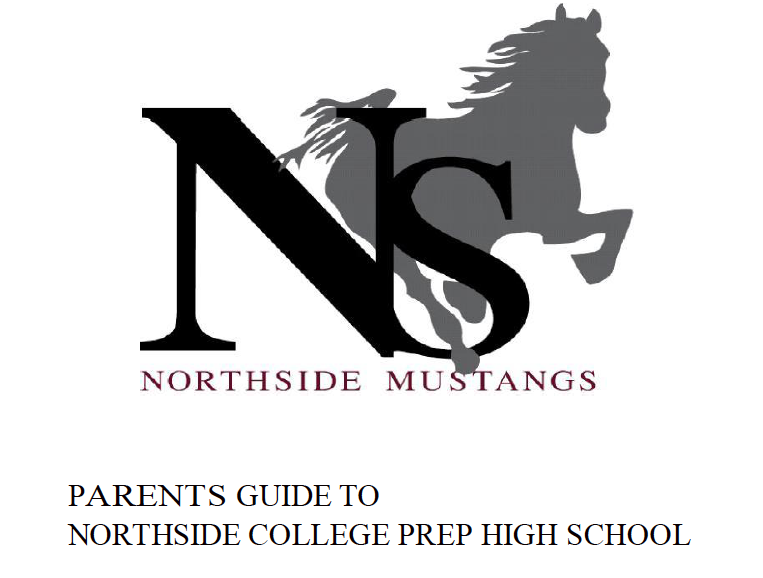# Navigating Northside

Navigating Northside, our parent handbook, is available on the school website under the Parents tab. Click HERE to access it directly.

 table div table+table+table+table+table+table+table+table+table+table+table+table+table+table+table+table+table+table+table+table+table+table+table+table+table+table+table+table+table+table+table+table+table+table+table+table+table+table+table+table+table+table+table+table+table+table+table+table+table+table+table+table+table+table+table+table+table+table+table+table+table+table+table+table+table+table+table+table+table+table+table+table div table{width:100%;padding:0}table div table+table+table+table+table+table+table+table+table+table+table+table+table+table+table+table+table+table+table+table+table+table+table+table+table+table+table+table+table+table+table+table+table+table+table+table+table+table+table+table+table+table+table+table+table+table+table+table+table+table+table+table+table+table+table+table+table+table+table+table+table+table+table+table+table+table+table+table+table+table+table+table div table img{width:96.23%;padding:0;float:none}table div table+table+table+table+table+table+table+table+table+table+table+table+table+table+table+table+table+table+table+table+table+table+table+table+table+table+table+table+table+table+table+table+table+table+table+table+table+table+table+table+table+table+table+table+table+table+table+table+table+table+table+table+table+table+table+table+table+table+table+table+table+table+table+table+table+table+table+table+table+table+table+table div table td{width:100%;padding:0 1.88% 18px}/* styles */# February College Visits at Northside

Thursday, February 28
Greenville University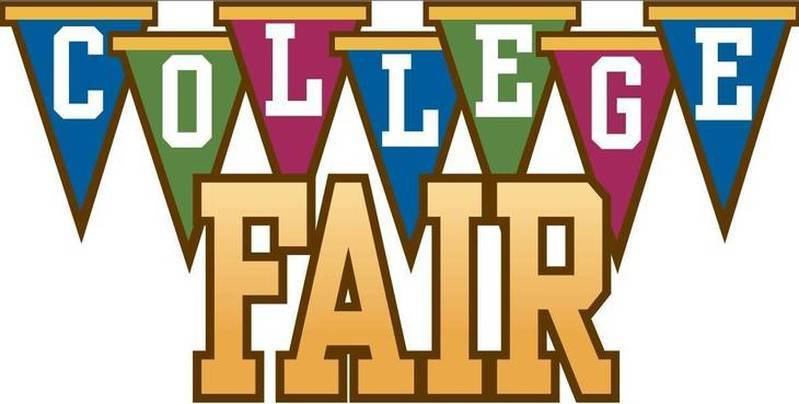# College Fair

Tuesday, March 5
6:00 - 8:00 pm
at NCP

Northside Prep, Disney II Magnet, and Von Steuben High School are hosting a joint College Fair.

Register today at www.strivefair.com.
If you have questions, contact Monique Moore, NCP College Counselor, mcmoore6@cps.edu or (773)-534-3954 x26811.

# CPS Scholarships and Non-NCP Events

For more information about general CPS scholarships and post-secondary events outside of Northside College Prep feel free to visit the NCP College Counseling Calendar HERE.

# SAT Prep Course

Bring sharpened pencils and a calculator for every class.

For more information, contact Monique Moore, Northside Prep College Counselor at mcmoore6@cps.edu or (773) 524-3954 x26811.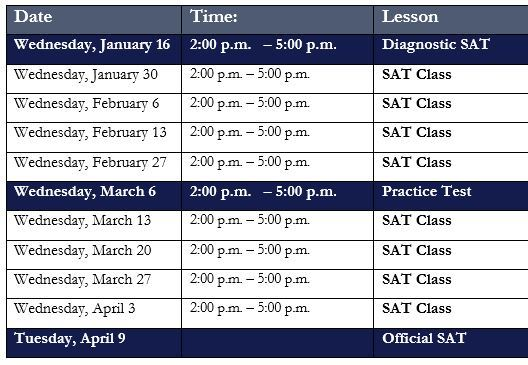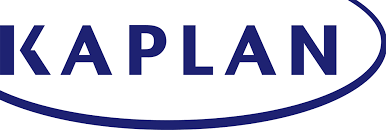# Naviance CCLR Live by Kaplan

## College, Career, and Life Readiness# NCP College Planning Handbook

This is a great resource that guides students through the entire college process. Click HERE to access a copy.

# NCP College Planning Additional Resources

Click on the links below to check out the additional resources on the Northside College Prep website:

 table div table+table+table+table+table+table+table+table+table+table+table+table+table+table+table+table+table+table+table+table+table+table+table+table+table+table+table+table+table+table+table+table+table+table+table+table+table+table+table+table+table+table+table+table+table+table+table+table+table+table+table+table+table+table+table+table+table+table+table+table+table+table+table+table+table+table+table+table+table+table+table+table+table+table+table+table+table+table+table+table+table+table div table{width:100%;padding:0}table div table+table+table+table+table+table+table+table+table+table+table+table+table+table+table+table+table+table+table+table+table+table+table+table+table+table+table+table+table+table+table+table+table+table+table+table+table+table+table+table+table+table+table+table+table+table+table+table+table+table+table+table+table+table+table+table+table+table+table+table+table+table+table+table+table+table+table+table+table+table+table+table+table+table+table+table+table+table+table+table+table+table div table img{width:96.23%;padding:0;float:none}table div table+table+table+table+table+table+table+table+table+table+table+table+table+table+table+table+table+table+table+table+table+table+table+table+table+table+table+table+table+table+table+table+table+table+table+table+table+table+table+table+table+table+table+table+table+table+table+table+table+table+table+table+table+table+table+table+table+table+table+table+table+table+table+table+table+table+table+table+table+table+table+table+table+table+table+table+table+table+table+table+table+table div table td{width:100%;padding:0 1.88% 18px}/* styles */# Help Northside When You Shop Online

## You can help our school with no extra cost to you.

AmazonSmile is a simple and automatic way to support Northside while you shop.

To choose Northside, please note that our AmazonSmile name is Friends of "North" "Side" (North Side - two words at AmazonSmile).

 table div table+table+table+table+table+table+table+table+table+table+table+table+table+table+table+table+table+table+table+table+table+table+table+table+table+table+table+table+table+table+table+table+table+table+table+table+table+table+table+table+table+table+table+table+table+table+table+table+table+table+table+table+table+table+table+table+table+table+table+table+table+table+table+table+table+table+table+table+table+table+table+table+table+table+table+table+table+table+table+table+table+table+table+table+table div table{width:100%;padding:0}table div table+table+table+table+table+table+table+table+table+table+table+table+table+table+table+table+table+table+table+table+table+table+table+table+table+table+table+table+table+table+table+table+table+table+table+table+table+table+table+table+table+table+table+table+table+table+table+table+table+table+table+table+table+table+table+table+table+table+table+table+table+table+table+table+table+table+table+table+table+table+table+table+table+table+table+table+table+table+table+table+table+table+table+table+table div table img{width:96.23%;padding:0;float:none}table div table+table+table+table+table+table+table+table+table+table+table+table+table+table+table+table+table+table+table+table+table+table+table+table+table+table+table+table+table+table+table+table+table+table+table+table+table+table+table+table+table+table+table+table+table+table+table+table+table+table+table+table+table+table+table+table+table+table+table+table+table+table+table+table+table+table+table+table+table+table+table+table+table+table+table+table+table+table+table+table+table+table+table+table+table div table td{width:100%;padding:0 1.88% 18px}/* styles */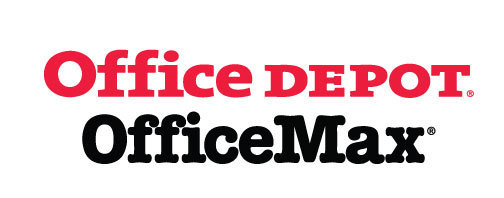# Give Back to Northside

Purchase school and office supplies at Office Depot and OfficeMax, and Northside can receive 5% back in credits or FREE supplies. Simply provide our school ID 70031171 at checkout, online or in stores. There’s no cost to you!

 table div table+table+table+table+table+table+table+table+table+table+table+table+table+table+table+table+table+table+table+table+table+table+table+table+table+table+table+table+table+table+table+table+table+table+table+table+table+table+table+table+table+table+table+table+table+table+table+table+table+table+table+table+table+table+table+table+table+table+table+table+table+table+table+table+table+table+table+table+table+table+table+table+table+table+table+table+table+table+table+table+table+table+table+table+table+table+table+table div table{width:100%;padding:0}table div table+table+table+table+table+table+table+table+table+table+table+table+table+table+table+table+table+table+table+table+table+table+table+table+table+table+table+table+table+table+table+table+table+table+table+table+table+table+table+table+table+table+table+table+table+table+table+table+table+table+table+table+table+table+table+table+table+table+table+table+table+table+table+table+table+table+table+table+table+table+table+table+table+table+table+table+table+table+table+table+table+table+table+table+table+table+table+table div table img{width:96.23%;padding:0;float:none}table div table+table+table+table+table+table+table+table+table+table+table+table+table+table+table+table+table+table+table+table+table+table+table+table+table+table+table+table+table+table+table+table+table+table+table+table+table+table+table+table+table+table+table+table+table+table+table+table+table+table+table+table+table+table+table+table+table+table+table+table+table+table+table+table+table+table+table+table+table+table+table+table+table+table+table+table+table+table+table+table+table+table+table+table+table+table+table+table div table td{width:100%;padding:0 1.88% 18px}/* styles */# Follow Us on Social Media

▪ Northside Prep Parents' Facebook Page, a closed group for parents, by parents, to share their knowledge of Northside
▪ Friends of Northside Facebook Page, for news everyone can view
 ▪ Northside Prep Parents' Facebook Page, a closed group for parents, by parents, to share their knowledge of Northside
 ▪ Friends of Northside Facebook Page, for news everyone can view
 table div table+table+table+table+table+table+table+table+table+table+table+table+table+table+table+table+table+table+table+table+table+table+table+table+table+table+table+table+table+table+table+table+table+table+table+table+table+table+table+table+table+table+table+table+table+table+table+table+table+table+table+table+table+table+table+table+table+table+table+table+table+table+table+table+table+table+table+table+table+table+table+table+table+table+table+table+table+table+table+table+table+table+table+table+table+table+table+table+table+table div table{width:100%;padding:0}table div table+table+table+table+table+table+table+table+table+table+table+table+table+table+table+table+table+table+table+table+table+table+table+table+table+table+table+table+table+table+table+table+table+table+table+table+table+table+table+table+table+table+table+table+table+table+table+table+table+table+table+table+table+table+table+table+table+table+table+table+table+table+table+table+table+table+table+table+table+table+table+table+table+table+table+table+table+table+table+table+table+table+table+table+table+table+table+table+table+table div table img{width:96.23%;padding:0;float:none}table div table+table+table+table+table+table+table+table+table+table+table+table+table+table+table+table+table+table+table+table+table+table+table+table+table+table+table+table+table+table+table+table+table+table+table+table+table+table+table+table+table+table+table+table+table+table+table+table+table+table+table+table+table+table+table+table+table+table+table+table+table+table+table+table+table+table+table+table+table+table+table+table+table+table+table+table+table+table+table+table+table+table+table+table+table+table+table+table+table+table div table td{width:100%;padding:0 1.88% 18px}/* styles */Northside’s website can be found HERE.

Friends of Northside's website can be found HERE.

 table div table+table+table+table+table+table+table+table+table+table+table+table+table+table+table+table+table+table+table+table+table+table+table+table+table+table+table+table+table+table+table+table+table+table+table+table+table+table+table+table+table+table+table+table+table+table+table+table+table+table+table+table+table+table+table+table+table+table+table+table+table+table+table+table+table+table+table+table+table+table+table+table+table+table+table+table+table+table+table+table+table+table+table+table+table+table+table+table+table+table+table+table div table{width:100%;padding:0}table div table+table+table+table+table+table+table+table+table+table+table+table+table+table+table+table+table+table+table+table+table+table+table+table+table+table+table+table+table+table+table+table+table+table+table+table+table+table+table+table+table+table+table+table+table+table+table+table+table+table+table+table+table+table+table+table+table+table+table+table+table+table+table+table+table+table+table+table+table+table+table+table+table+table+table+table+table+table+table+table+table+table+table+table+table+table+table+table+table+table+table+table div table img{width:96.23%;padding:0;float:none}table div table+table+table+table+table+table+table+table+table+table+table+table+table+table+table+table+table+table+table+table+table+table+table+table+table+table+table+table+table+table+table+table+table+table+table+table+table+table+table+table+table+table+table+table+table+table+table+table+table+table+table+table+table+table+table+table+table+table+table+table+table+table+table+table+table+table+table+table+table+table+table+table+table+table+table+table+table+table+table+table+table+table+table+table+table+table+table+table+table+table+table+table div table td{width:100%;padding:0 1.88% 18px}/* styles */